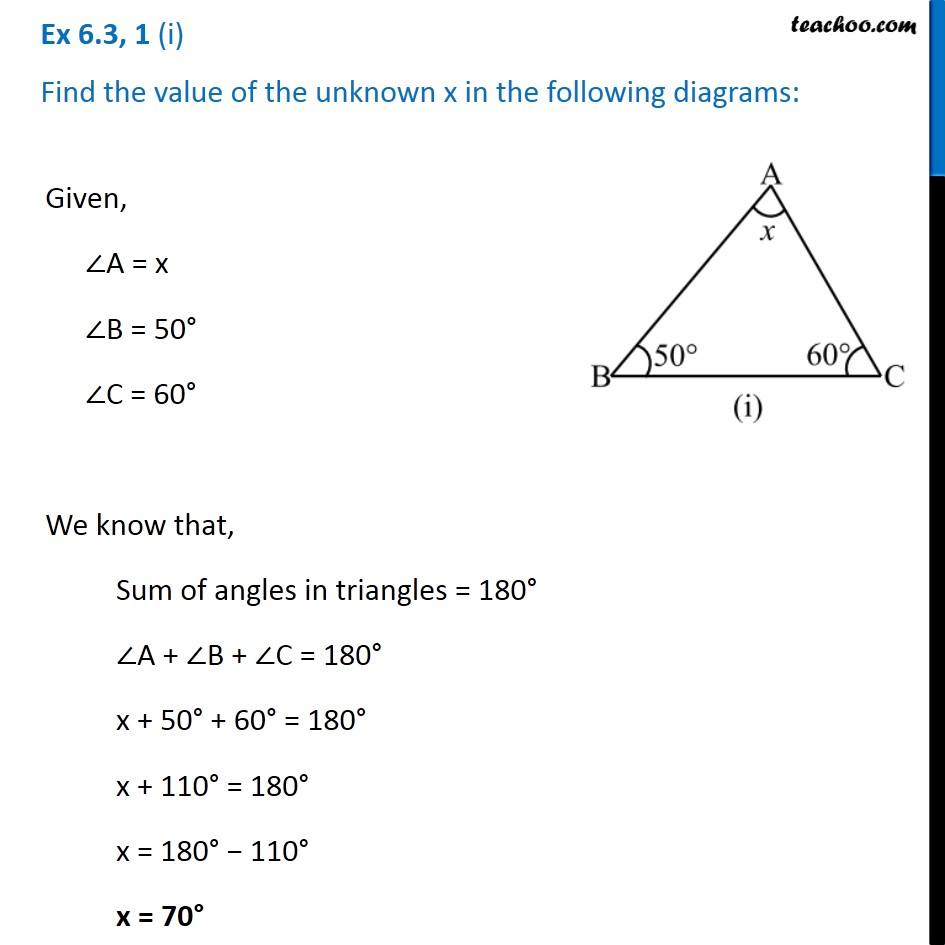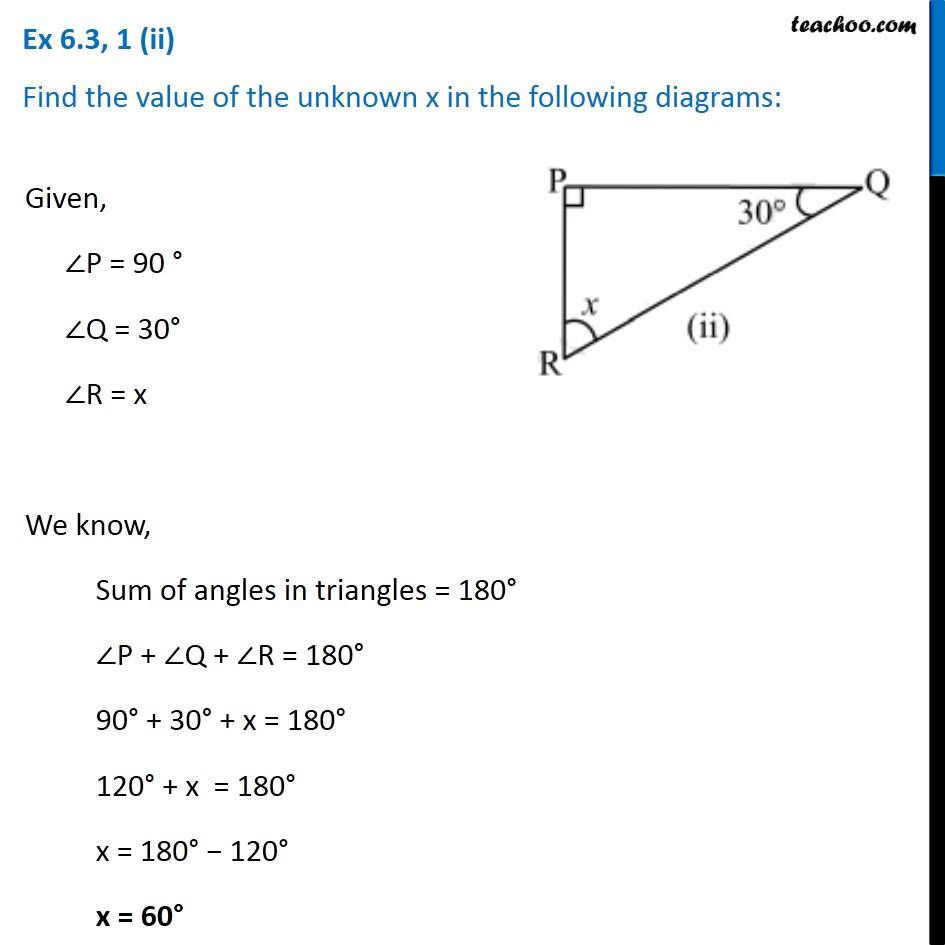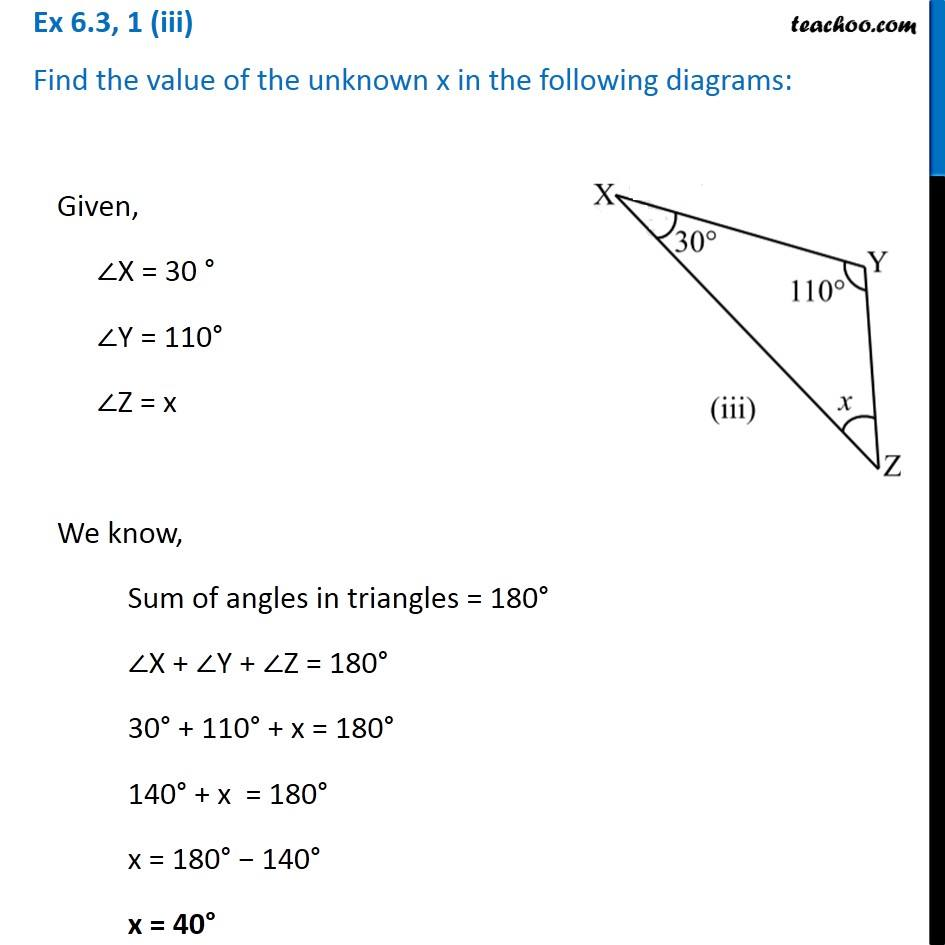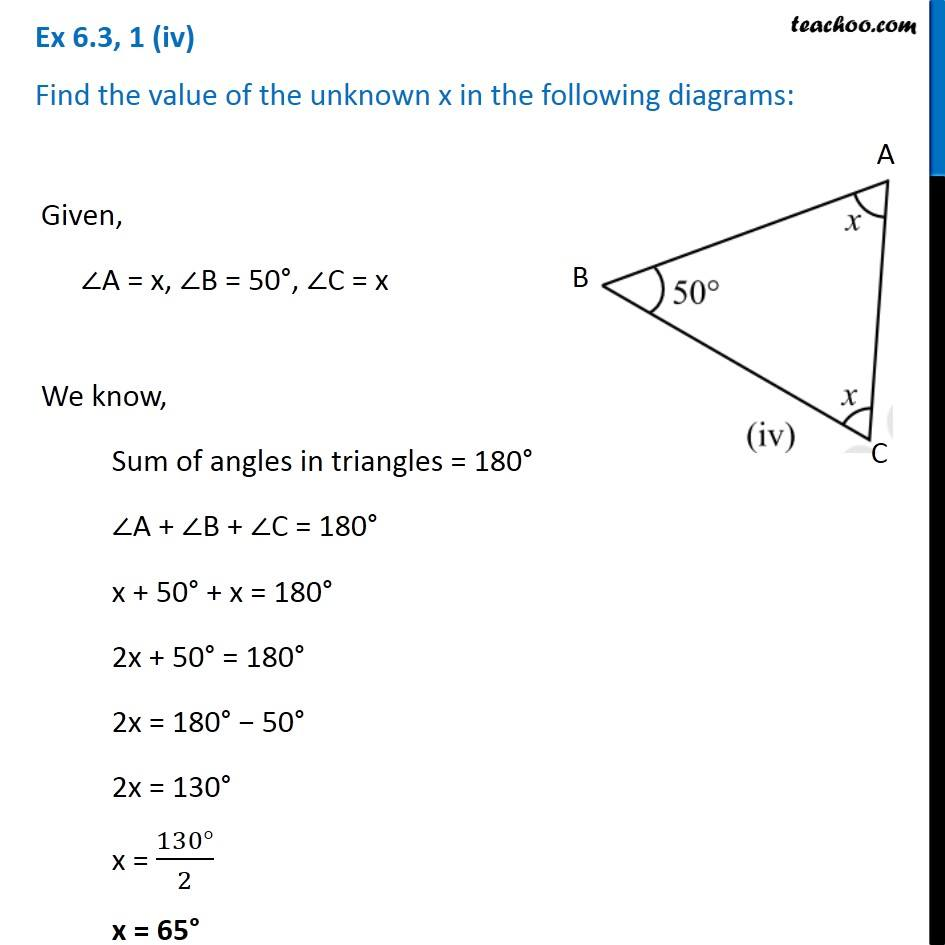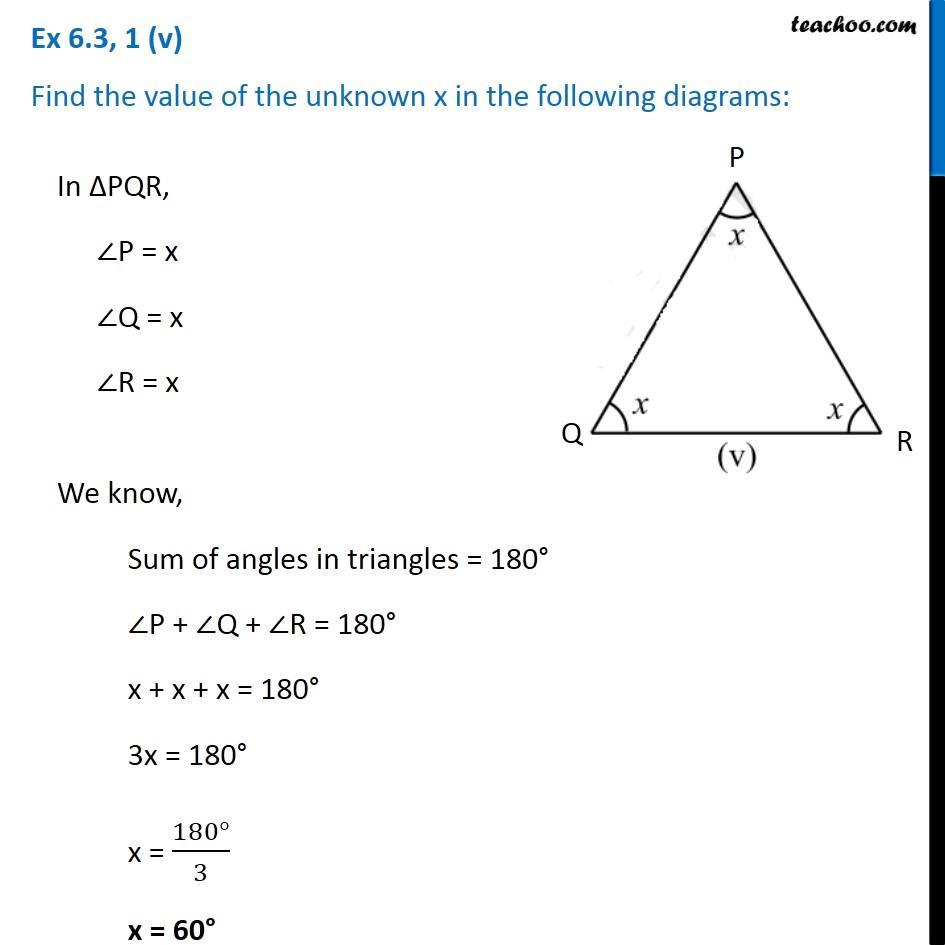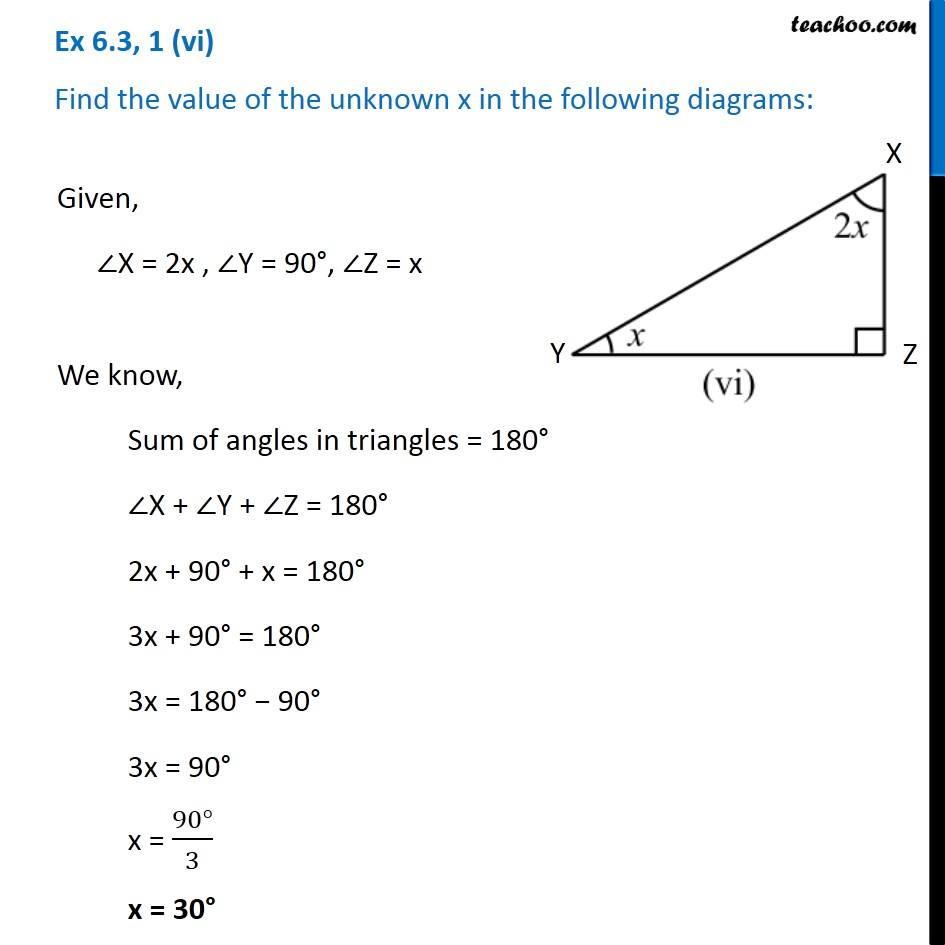Subscribe to our Youtube Channel - https://you.tube/teachoo

1. Chapter 6 Class 7 Triangle and its Properties
2. Concept wise
3. Angle sum property of a triangle

Transcript

Ex 6.3, 1 (i) Find the value of the unknown x in the following diagrams: Given, ∠A = x ∠B = 50° ∠C = 60° We know that, Sum of angles in triangles = 180° ∠A + ∠B + ∠C = 180° x + 50° + 60° = 180° x + 110° = 180° x = 180° − 110° x = 70° Ex 6.3, 1 (ii) Find the value of the unknown x in the following diagrams: Given, ∠P = 90 ° ∠Q = 30° ∠R = x We know, Sum of angles in triangles = 180° ∠P + ∠Q + ∠R = 180° 90° + 30° + x = 180° 120° + x = 180° x = 180° − 120° x = 60° Ex 6.3, 1 (iii) Find the value of the unknown x in the following diagrams: Given, ∠X = 30 ° ∠Y = 110° ∠Z = x We know, Sum of angles in triangles = 180° ∠X + ∠Y + ∠Z = 180° 30° + 110° + x = 180° 140° + x = 180° x = 180° − 140° x = 40° Ex 6.3, 1 (iv) Find the value of the unknown x in the following diagrams: Given, ∠A = x, ∠B = 50°, ∠C = x We know, Sum of angles in triangles = 180° ∠A + ∠B + ∠C = 180° x + 50° + x = 180° 2x + 50° = 180° 2x = 180° − 50° 2x = 130° x = (130°)/2 x = 65° Ex 6.3, 1 (v) Find the value of the unknown x in the following diagrams:In ∆PQR, ∠P = x ∠Q = x ∠R = x We know, Sum of angles in triangles = 180° ∠P + ∠Q + ∠R = 180° x + x + x = 180° 3x = 180° x = (180°)/3 x = 60° Ex 6.3, 1 (vi) Find the value of the unknown x in the following diagrams: Given, ∠X = 2x , ∠Y = 90°, ∠Z = x We know, Sum of angles in triangles = 180° ∠X + ∠Y + ∠Z = 180° 2x + 90° + x = 180° 3x + 90° = 180° 3x = 180° − 90° 3x = 90° x = (90°)/3 x = 30°

Angle sum property of a triangle